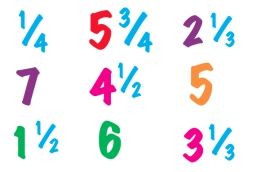# Lowest terms

What is the sum if the product of 1 3/4 and 2 1/6 is added to the quotient of 1/3 and 8 4/5? Write the solution as a mixed number or a fraction in the lowest terms.

s =  30 23120 = 3623/120

### Step-by-step explanation:Did you find an error or inaccuracy? Feel free to write us. Thank you!

Tips for related online calculators
Need help calculating sum, simplifying, or multiplying fractions? Try our fraction calculator.
Need help with mixed numbers? Try our mixed-number calculator.